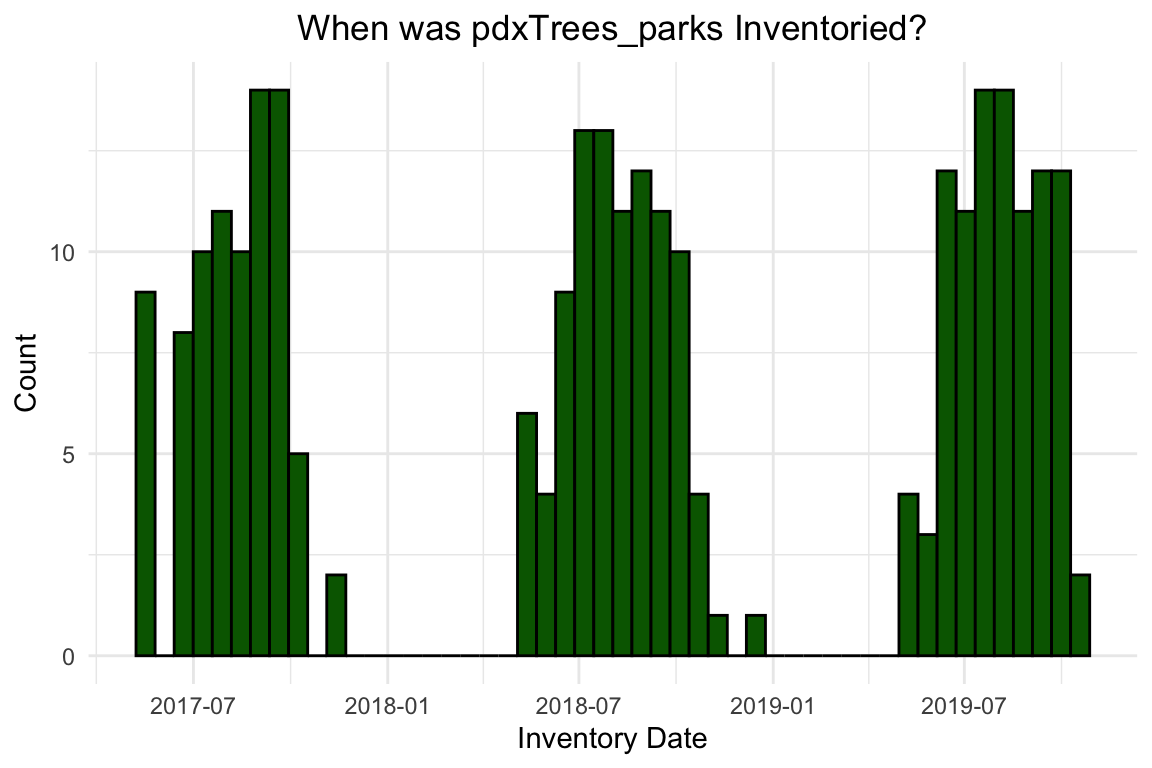# The Many Uses of pdxTrees

`pdxTrees` is a data package composed of information on inventoried trees in Portland, OR. There are two datasets that can be accessed with this package:

• `get_pdxTrees_parks()` pulls in data on up to 25,534 trees from 174 Portland parks.

• `get_pdxTrees_streets()` pulls in data on up to 218,602 trees located on Portland’s streets. A street tree is loosely defined as a tree generally in the public right-of-way, usually between the sidewalk and the street.

The street trees are categorized by one of the 96 Portland neighborhoods and the park trees are categorized by the public parks in which they grow.

Here are some examples of the different ways `pdxTrees` can be used in an educational setting!

``````# First make sure you have the package downloaded!

# devtools::install_github("mcconvil/pdxTrees")

# Loading the required libraries

library(pdxTrees)
library(ggplot2)
library(dplyr)
library(forcats)``````

First we have to grab the data. To do this we use the `get_pdxTrees_parks()` and `get_pdxTrees_streets()` functions. In this vignette, we only explore the parks dataset.

``````
# Leaving the argument field blank pulls data for all of the parks!

pdxTrees_parks <- get_pdxTrees_parks()``````

## Graphing with `ggplot2`

``````
# A histogram of the inventory date
pdxTrees_parks %>%
count(Inventory_Date) %>%
# Setting the aesthetics
ggplot(aes(x = Inventory_Date)) +
# Specifying a histogram and picking color!
geom_histogram(bins = 50,
fill = "darkgreen",
color = "black") +
labs( x = "Inventory Date",
y = "Count",
title= "When was pdxTrees_parks Inventoried?") +
# Adding a theme
theme_minimal() +
theme(plot.title = element_text(hjust = 0.5))``````Using `ggplot2` we can create a histogram of the `pdxTrees_parks` inventory dates. The trees were inventoried from 2017 to 2019 with the majority of the trees inventoried in the summer months, when the weather is nice in Portland.

This graph is just one of example of how `pdxTrees` can be used to create data visualizations. With a healthy mix of categorical and quantitative variables in both datasets, you can make scatterplots, bar graphs, density plots, etc. For more advanced visualizations, you can add animation with `gganimate` or create an interactive map with `leaflet`.

## An interactive map with `leaflet`

The following code creates an interactive map with `leaflet` and the `pdxTrees_parks` data. It showcases:

• Adding popups for each tree where you can customize the displayed information.
• Changing the background map.
• Including a mini-map.
``````# Loading the leaflet packages
library(leaflet)
library(leaflet.extras)``````
``````
# Making the leaf popup icon
greenLeaflittle <- makeIcon(
iconUrl = "http://leafletjs.com/examples/custom-icons/leaf-green.png",
iconWidth = 10, iconHeight = 20,
iconAnchorX = 10, iconAnchorY = 10,
shadowUrl = "http://leafletjs.com/examples/custom-icons/leaf-shadow.png",
shadowWidth = 10, shadowHeight = 15,
shadowAnchorX = 5, shadowAnchorY = 5
)

# Pulling the data for Berkely Park

berkeley_prk <- get_pdxTrees_parks(park = "Berkeley Park")

# Creating the popup label

labels <- paste("</b>", "Common Name:",
berkeley_prk\$Common_Name,
"</b></br>", "Factoid: ",
berkeley_prk\$Species_Factoid)

# Creating the map

leaflet() %>%
# Setting the lng and lat to be in the general area of Berekely Park
setView(lng = -122.6239, lat = 45.4726, zoom = 17) %>%
# Setting the background tiles
addProviderTiles(providers\$Esri.WorldTopoMap) %>%
# Adding the leaf markers with the popup data on top of the circles markers
addMarkers( ~Longitude, ~Latitude,
data = berkeley_prk,
icon = greenLeaflittle,
popup = ~labels) %>%
# Adding the mini map at the bottom right corner
addMiniMap()``````

## Creating an animated graph using `gganimate`

``library(gganimate)``

Before you animate a graph with `gganimate` you have to create and save a graph with `ggplot2`.

``````
# Refactoring the categorical mature_size variable
berkeley_prk <- berkeley_prk %>%
mutate(mature_size = fct_relevel(Mature_Size, "S", "M", "L"))

# First creating the graph using ggplot and saving it!
berkeley_graph <- berkeley_prk %>%
# Piping in the data
ggplot(aes(x = Tree_Height,
y = Pollution_Removal_value,
color = Mature_Size)) +
# Creating the scatterplot
geom_point(size = 2, alpha = 0.5) +
theme_minimal() +
# Adding the labels
labs(title = "Pollution Removal Value of
Berkeley Park Trees",
x = "Tree Height",
y = "Pollution Removal Value (\$'s annually)",
color = "Mature Size") +
# Adding a color palette
scale_color_brewer(type = "seq", palette = "Set1") +
# Customizing the title font
theme(plot.title = element_text(hjust = 0.5,
size = 8,
face = "bold"),
axis.title.x = element_text(size = 6),
axis.text = element_text(size = 4),
axis.title.y = element_text(size = 6),
legend.title = element_text(size = 6),
legend.text = element_text(size= 4))``````

Now we can add animation!

``````# Then adding the animation with gganimate functions

berkeley_graph +
# Choosing which variable we want to annimate
transition_states(states = Mature_Size,
# How long each point stays before fading away
transition_length = 10,
# Time the transition takes
state_length = 8)  +
# Animation for the points entering
enter_grow() +
# Animation for the points exiting
exit_shrink()``````